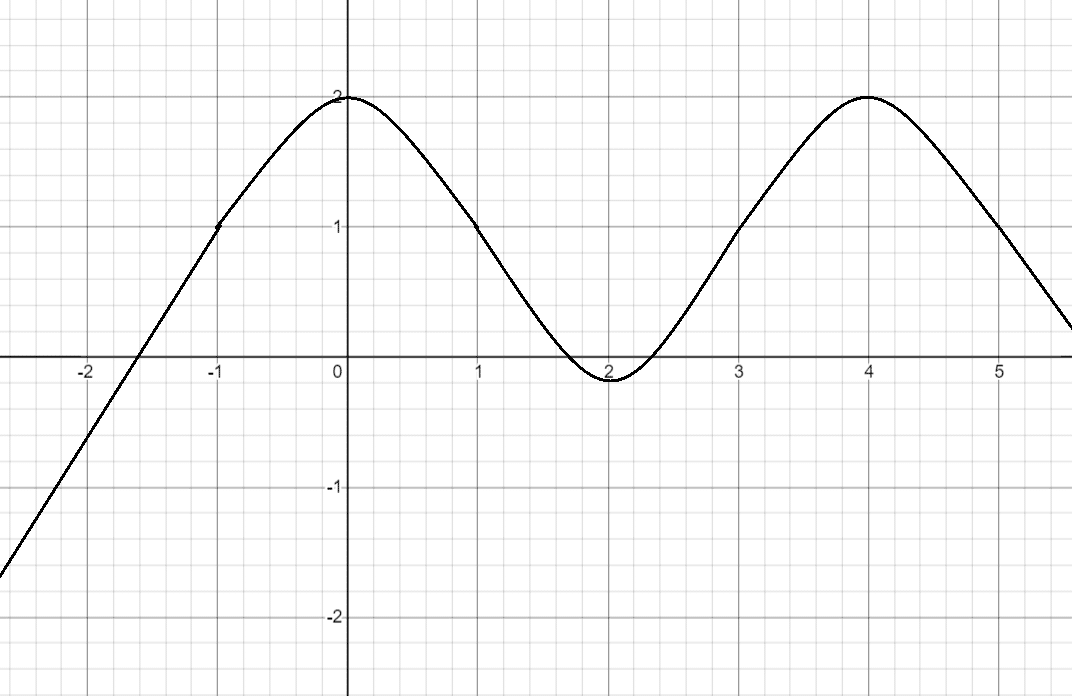# Sketch The Graph Of A Function That Satisfies All Of The Given Conditions

We thoroughly check each answer to a question to provide you with the most correct answers. Found a mistake? Let us know about it through the REPORT button at the bottom of the page.

Sketch the graph of a function that satisfies all of the given conditions.f'(0)=f'(2)=f'(4)=0, f'(x)>0 if x<0 or 2<x<4, f'(x)<0 if 0<x<2 or x>4, f”(x)>0 if 1<x<3, f”(x)<0 if x<1 or x>3

Answer: f has the local maximum values at x=0 and x=4, and it has a local minimum value at x=2.

## Explanation

f is increasing when x < 0 and 2 < x < 4;

f is decreasing when 0 < x < 2 and x > 4;

f has local minimum at x=2 and local maximum at x=0 and x=4 because of the way it shifts between increasing and decreasing.

f is concave up when 1 < x < 3 and concave down when x < 1 and x > 3.

It has inflection points at x=1 and x=3.